# User:IssaRice/Linear algebra/Type checking vector spaces

If$V$ is an arbitrary vector space, it does not in general make sense to ask whether$v \in V$ is a string of numbers. This is because we have not chosen a coordinate system.
If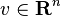$v \in \mathbf R^n$ and$a_1,\ldots,a_n \in \mathbf R$, then it does make sense to ask whether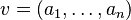$v = (a_1,\ldots,a_n)$.
If$V$ is an arbitrary finite-dimensional real vector space,$\beta = (v_1, \ldots, v_n)$ is a basis for$V$, and$a_1,\ldots,a_n \in \mathbf R$, then it does make sense to ask whether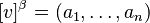$[v]^\beta = (a_1,\ldots,a_n)$, or equivalently, to ask whether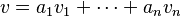$v = a_1v_1 + \cdots + a_nv_n$.
If$v \in \mathbf R^n$ and$\beta = (v_1, \ldots, v_n)$ is a basis for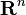$\mathbf R^n$, then$v$ and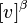$[v]^\beta$ have the same type, so it makes sense to ask whether$v = [v]^\beta$. When are the two equal? If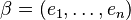$\beta = (e_1, \ldots, e_n)$ is the standard basis and$v = (a_1, \ldots, a_n)$, then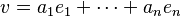$v = a_1e_1 + \cdots + a_ne_n$ so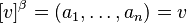$[v]^\beta = (a_1, \ldots, a_n) = v$. But the converse is not true: given$v = [v]^\beta$, there can be many bases that give the same coordinates. For instance if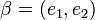$\beta = (e_1, e_2)$ and$\beta' = (e_2, e_1)$, and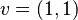$v=(1,1)$, then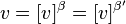$v = [v]^\beta = [v]^{\beta'}$.